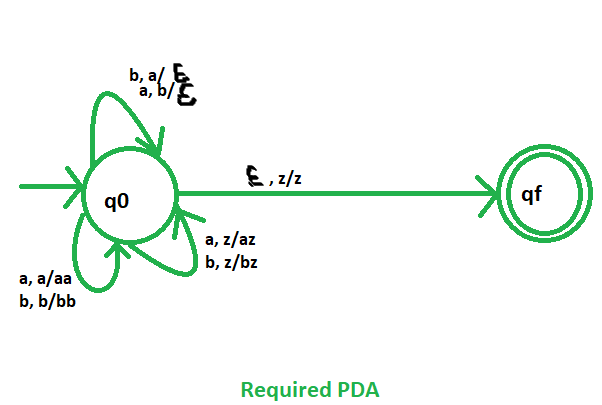# NPDA for the language L ={w∈ {a,b}*| w contains equal no. of a’s and b’s}

Prerequisite – Pushdown automata, Pushdown automata acceptance by final state
Problem – Design a non deterministic PDA for accepting the language L ={w?{a,b}* | w contains equal no. of a’s and b’s}, i.e.,

L = {ab, aabb, abba, aababb, bbabaa, baaababb, .......}

The number of a’s and b’s are same in all the strings.

Explanation –
Here, we need not to maintain any order of a’s and b’s. Thus our state diagram will contain only an initial state and a final state. The count of a’s and b’s is maintained by stack. We will take 3 stack alphabets:= {a, b, z}

Where,= set of all the stack alphabet
z = stack start symbol

Approach used in the construction of PDA –
If ‘a’ comes first then push it in stack and if again ‘a’ comes then also push it. Similarly, if ‘b’ comes first (‘a’ did not comes yet) then push it into the stack and if again ‘b’ comes then also push it.

Now, if ‘a’ is present in the top of the stack and ‘b’ comes then pop the ‘a’ from the stack. And if ‘b’ present in the top of the stack and ‘a’ comes then pop the ‘b’ from the stack.

So, at the end if the stack becomes empty then we can say that the string is accepted by the PDA.

Stack transition functions –(q0, a, z)(q0, az)(q0, a, a)(q0, aa)(q0, b, z)(q0, bz)(q0, b, b)(q0, bb)(q0, a, b)(q0,)(q0, b, a)(q0,)(q0,, z)(qf, z)


Where, q0 = Initial state
qf = Final state= indicates pop operationSo, this is our required non deterministic PDA for accepting the strings which contain equal no. of a’s and b’s.
Example:We will take one input string: “aabbba” PDA accept or not?.
solution:
1.Scan string from left to right.
2.on input ‘a’ and STACK alphabet Z, push the ‘a’s into STACK as : (a,Z/aZ) and state will be q0.
3.second input ‘a’ and STACK alphabet ‘a’, push the ‘a’s into STACK as : (a,a/aa) and state will be q0.
4.Third input ‘b’ and STACK alphabet ‘a’, pop from STACK as : (b,a/∈) and state will be q0.
5.on input ‘b’ and STACK alphabet ‘a’, pop from STACK as : (b,a/∈) and state will be q0.
6.on input ‘b’and STACK alphabet Z, push the ‘b’s into STACK as : (b,Z/bZ) and state will be q0.
7.on input ‘a’ and STACK alphabet ‘b’, pop from STACK as : (a,b/∈) and state will be q0.
8.on input ∈ and STACK alphabet Z, go to final state(qf) as : (∈, Z/Z).

So, at the end the stack becomes empty then we can say that the string is accepted by the PDA.My Personal Notes arrow_drop_upCheck out this Author's contributed articles.

If you like GeeksforGeeks and would like to contribute, you can also write an article using contribute.geeksforgeeks.org or mail your article to contribute@geeksforgeeks.org. See your article appearing on the GeeksforGeeks main page and help other Geeks.

Please Improve this article if you find anything incorrect by clicking on the "Improve Article" button below.

Improved By : Rumor

Article Tags :

Be the First to upvote.

Please write to us at contribute@geeksforgeeks.org to report any issue with the above content.